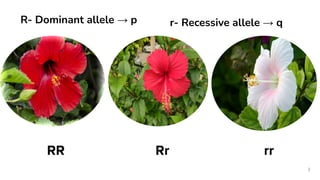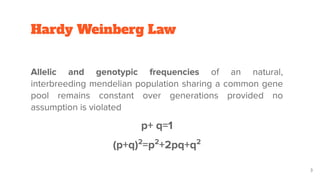O slideshow foi denunciado.
Seu SlideShare está sendo baixado. ×

# Hardy Weinberg lawCarregando em…3
×

1 de 24 Anúncio
1 de 24 Anúncio

# Hardy Weinberg law

Hardy Weinberg law
Hardy Weinberg Equilibrium with Solved Questions|CSIR NET|Life Sciences|GATE|JRF|ICMR|

Hardy Weinberg Law along with the assumptions the law is based on. Calculation of allelic and genotypic frequencies. Application of Hardy Weinberg law to different cases viz Multiple alleles, Polyploidy, Inbreeding and X-linked + Questions are discussed.

Hardy Weinberg law
Hardy Weinberg Equilibrium with Solved Questions|CSIR NET|Life Sciences|GATE|JRF|ICMR|

Hardy Weinberg Law along with the assumptions the law is based on. Calculation of allelic and genotypic frequencies. Application of Hardy Weinberg law to different cases viz Multiple alleles, Polyploidy, Inbreeding and X-linked + Questions are discussed.

Anúncio
Anúncio

### Hardy Weinberg law

1. 1. HARDY WEINBERG EQUILIBRIUM R r
2. 2. 2 RR Rr rr R- Dominant allele → p r- Recessive allele → q
3. 3. Hardy Weinberg Law Allelic and genotypic frequencies of an natural, interbreeding mendelian population sharing a common gene pool remains constant over generations provided no assumption is violated p+ q=1 (p+q)2 =p2 +2pq+q2 3
4. 4. ASSUMPTIONS 4 Random Mating No natural selection No mutation No migration Large population size Equal opportunity of mating with any other individual of the population- ‘panmictic population’ No individual will have a reproductive advantage over another because of its genotype Random ﬂuctuation in allele frequency-Genetic drift
5. 5. Hardy Weinberg Law Relates allelic and genotypic frequencies on an natural, interbreeding mendelian population sharing a common gene pool Rule has 3 aspects 5 Allelic Frequency Equilibrium Genotypic Frequency Equilibrium Neutral Equilibrium
6. 6. 6 RR Rr rr
7. 7. Calculating frequencies Allelic frequencies p= f(R) = 2 x number of RR+ number of Rr 2 X Total number = f(RR)+ ½ f(Rr) q= f(rr)+ ½ f(Rr) Genotypic frequencies(Individuals) RR: p2 Rr: 2pq rr: q2 (p+q)2 =p2 +2pq+q2 =1 p+q=1 7 Homozygous or Heterozygous
8. 8. EXAMPLE In a population frequency of a homozygous recessive disease is 16% then the frequency of dominant allele would be? a. 0.84 b. 0.6 c. 0.1 d. 0.4 8 q2 = 16%= 16/100=0.16 q= 0.4 p=1-q=1-0.4=0.6
9. 9. EXAMPLE In a population obeying Hardy-Weinberg equilibrium, the frequency of recessive allele is 0.88, while of dominant allele is 0.12. The frequency of heterozygotes in population will be? a. 11.1% b. 21.1% c. 79.9% d. 14.4% 9 q=0.88 p=0.12 2pq= 2 x 0.88 x 0.12 =21.12
10. 10. Question (June 2006) If the frequency of recessive allele causing disease in homozygous recessive condition in a population of 10,000 is 0.04, then the number of people affected by disease will be? a. 16 b. 400 c. 3600 d. 496 10 q= 0.04 q2 = 0.0016 Affected= q = 0.0016 x 10000= 16
11. 11. MULTIPLE ALLELES INBREEDING SEX LINKED POLYPLOIDY
12. 12. CASES Multiple alleles (p+q+r)2 =p2 +q2 +r2 +2pq+2qr+2p r Eg. Blood groups 12 Blood types Genotype Frequency A IA IA + IA i p2 + 2pr B IB IB + IB i q2 + 2qr AB IA IB 2pq O ii r2
13. 13. EXAMPLE In a large randomly mating human population, the frequencies of the IA ,IB and i alleles are 0.7, 0.2 and 0.1, respectively. Calculate the expected frequencies for each blood type Let the Three alleles be p, q and r Since population is in Hardy Weinberg population, genotypic frequencies can be calculated as: (p+q+r)2 = p2 +q2 +2pq+2pr+2qr Let, f(IA )=p=0.7 f(IB )=q=0.2 f(i)=r=0.1
14. 14. Blood type A = IA IA + IA i p2 + 2pr= 0.49+0.14=0.63 B = IB IB + IB i q2 +2qr =0.04+0.04=0.08 AB= IA IB 2pq=2x 0.7 x 0.2=0.28 O= ii r2 =0.01 Let, f(IA )=p=0.7 f(IB )=q=0.2 f(i)=r=0.1
15. 15. CASES Polyploidy (p+q)n n=polyploidy 15 Diploid (p+q)2 = p2 +2pq+q2 Triploid (p+q)3 = p3 + q3 + 3p2 q+3q2 p Tetraploid(p+q)4 = p4 +4p3 q+6p2 q2 +4pq3 +q4
16. 16. June 2007 If an organism is triploid, then Hardy-weinberg theorem applicable will be? a. (p+q)3 b. (p+q)2 c. (p+q+r)3 d. (p+q+r)2 16
17. 17. 17 Fruit colour of wild Solanum nigrum is controlled by two alleles of a gene (A and a). The frequency of A, p=0.8 and a, q=0.2. In a neighbouring ﬁeld a tetraploid genotype of S.nigrum was found. After critical examination ﬁve distinct genotypes found; which are AAAA, AAAa, AAaa and aaaa. Following Hardy- Weinberg principle and assuming the same allelic frequency as that of diploid population the numbers of phenotypes calculated within a population of 1000 plants are close to one of the following: AAAA: AAAa: AAaa: Aaaa: aaaa a. 409:409:154:26.2 b. 420:420:140:18.2 c. 409:409:144:36.2 d. 409:420:144:25.2
18. 18. 18 Fruit colour controlled by two alleles frequency of A, p=0.8 and a, q=0.2 Neighbouring ﬁeld a tetraploid genotype was found having ﬁve distinct genotypes AAAA, AAAa, AAaa and aaaa. Numbers of phenotypes calculated within a population of 1000 plants are close to one of the following: AAAA: AAAa: AAaa: Aaaa: aaaa Tetraploid(p+q)4 = p4 +4p3 q+6p2 q2 +4pq3 +q4 f(AAAA)= p4 =(0.8)4 =0.4096; out of 1000 plants- 409.6 f(AAAa)=4p3 q=4x(0.8)3 x0.2=0.4096 f(AAaa)=6p2 q2 = 6x(0.8)2 x(0.2)2 =0.1536 a. 409:409:154:26.2 b. 420:420:140:18.2 c. 409:409:144:36.2 d. 409:420:144:25.2
19. 19. Sex linked genes Male= Heterogametic Thus will need only 1 copy of recessive allele to have the disease Frequency will be equal to 19 Genotype Genotype frequency XA Y p XB Y q Female= Homogametic They will need both copies of the allele to be affected Frequency will be equal to Genotype Genotype frequency XA XA p2 XA Xa 2pq Xa Xa q2
20. 20. Example Frequency of recessive allele for an X-linked recessive disease in human population is 0.02. Calculate proportion of diseased individuals 20 MALES: 1 copy needed Frequency of diseased male=frequency of recessive allele =0.02 FEMALES: Both copies needed Frequency of diseased=q2 =(0.02)2 =0.0004 Overall frequency(if equal males & females) =½ x 0.0.2+½ x 0.0004 =0.0102
21. 21. Inbreeding ● Mating between individuals that are closely related through common ancestry ● Extent of inbreeding can be measured by “inbreeding coefﬁcient” ● Reduction in heterozygote frequencies How? 21
22. 22. 22
23. 23. Inbreeding coefficient Relative reduction in heterozygosity in the population due to inbreeding Given by, F=2pq-H = 1- H 2pq 2pq 23
24. 24. DEC 2011 The frequencies of two alleles p and q for a gene locus in a population at Hardy Weinberg equilibrium are 0.3 and 0.7 respectively. After a few generations frequency was found to be 0.28. The inbreeding coefﬁcient in this case is: a. 0.42 b. 0.28 c. 0.33 d. 0.67 24 F= 1 -(H/2pq)= 1-(0.28/ 2x0.3x0.7)=1-(0.28/0.42)=1-0.67=0.33